## Example Questions

← Previous 1

### Example Question #1 : How To Find Decimal Equivalent To A Percentage

55 and 1/2% of 23 is about what?

11

2

13

155

49

13

Explanation:

55 and 1/2% can be written as a decimal: 0.555. To see what number is about 55.5% of 23, multiply 0.555 by 23. Answer: 12.765 or about 13.

Another route is to say that 55.5% is about half of 23. Half of 23 is 11.5. Since 55.5% is greater than 50%, 13 is the logical choice instead of 11.

### Example Question #1 : How To Find Decimal Equivalent To A Percentage

Let x and y be numbers such that x and y are both nonzero, and x > y. If half of x is equal to thirty percent of the positive difference between x and y, then what is the ratio of x to y?

–1

3/2

2/3

–3/2

–2/3

–3/2

Explanation:

We need to find expressions for fifty percent of x and for thirty percent of the positive difference between x and y. Then, we can set these two expressions equal to each other and determine the ratio of x to y.

Fifty percent of x is equal to one-half of x, which is the same as multiplying x by 0.50.

50% of x = 0.5x

Thirty percent of the positive difference between x and y means that we need to multiply the positive difference between x and y by thirty percent. Because x > y, the positive difference between x and y is equal to x – y. We then need to take thirty percent of the quantity x – y. Remember that to convert from a percent to a decimal, we move the decimal two spaces to the left. Therefore, 30% = 0.30. We can now multiply this by (x – y).

30% of x – y = 0.30(x – y)

Now, we set the two expressions equal to one another.

0.5x = 0.30(x – y)

Distribute the right side.

0.5x = 0.3x – 0.3y

The ratio of x to y is represent by x/y. Thus, we want to group the x and y terms on opposite sides of the equations, and then divide both sides by y.

0.5x = 0.3x – 0.3y

Subtract 0.3x from both sides.

0.2x = –0.3y

Divide both sides by 0.2

x = (–0.3/0.2)y

Divide both sides by y to find x/y.

x/y = (–0.3/0.2) = –1.5.

Because the answers are in fractions, we want to rewrite –1.5 as a fraction. We can write –1.5 as –1.5/1 and then mutiply the top and bottom by 2.

(–1.5/1)(2/2) = –3/2

### Example Question #41 : Percentage

If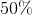ofis equal toof, andofis equal to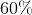of, then what percent ofis?

75

25

125

133

100

75

Explanation:

We are told that 50% of x is equal to 25% of y. We need to represent these two pieces of information as algebraic expressions. We can convert 50% and 25% to decimals by moving the decimals two places to the left. Thus, 50% = 0.50, and 25% = 0.25. To find 50% of x, we multiply x by 0.50. In other words, 50% of x = 0.50x. Likewise, 25% of y = 0.25y. We now set 0.50x and 0.25y equal to one another.

0.50x = 0.25y

Let's divide both sides by 0.25 to get rid of decimals.

2x = y

Next, we are told that 40% of y is equal to 60% of z. We will represent 40% and 60% as 0.40 and 0.60, respectively. Thus, we can write the following equation:

0.40y = 0.60z

Ultimately, we are asked to find x as a percentage of z. This means we want to find an equation with x and z, but not y. If we solve for y in the second equation, and then substitute this value into the first, we can eliminate y.

Let's take the equation 0.40y = 0.60z and divide both sides by 0.40.

y = 1.5z

Now, we can take 1.5z and substitute this for y in the first equation.

2x = 1.5z

In order to find x as a percent of z, we must solve for x in terms of z. This means we must divide both sides of the equation by 2.

x = 0.75z

x is 0.75 times z. We can represent 0.75 as 75%, because in order to convert from a decimal to a percent, we need to move the decimal two spaces to the right. Therefore, if x = 0.75z, then x = 75% of z.

### Example Question #211 : Arithmetic

What is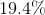in decimal form?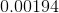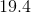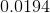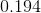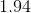Explanation:

The correct answer isThis can be obtained by taking the percentage ofand dividing by.

This shifts the decimal place over two places to the left, which results inas the decimal of.

### Example Question #5 : How To Find Decimal Equivalent To A Percentage

Find the decimal equivalent to the percentage: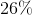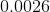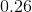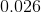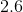Explanation:

In order to find the decimal equivalent of a percentage, the number that makes up the percent has to be divided by 100. However, since it is division by a power of 10, we can accomplish the same thing, by moving the decimal point 2 places to the left, thus making the number smaller. For this problem, that looks like this: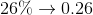### Example Question #6 : How To Find Decimal Equivalent To A Percentage

Find the decimal equivalent to the percentage: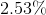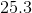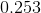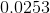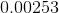Explanation:

In order to find the decimal equivalent of a percentage, the number that makes up the percent has to be divided by 100. However, since it is division by a power of 10, we can accomplish the same thing, by moving the decimal point 2 places to the left, thus making the number smaller. For this problem, that looks like this: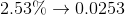### Example Question #7 : How To Find Decimal Equivalent To A Percentage

Find the decimal equivalent to the percentage: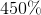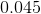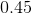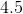Explanation:

In order to find the decimal equivalent of a percentage, the number that makes up the percent has to be divided by 100. However, since it is division by a power of 10, we can accomplish the same thing, by moving the decimal point 2 places to the left, thus making the number smaller. For this problem, that looks like this: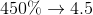### Example Question #8 : How To Find Decimal Equivalent To A Percentage

Find the decimal equivalent to the percentage: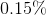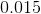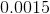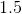Explanation:

In order to find the decimal equivalent of a percentage, the number that makes up the percent has to be divided by 100. However, since it is division by a power of 10, we can accomplish the same thing, by moving the decimal point 2 places to the left, thus making the number smaller. For this problem, that looks like this: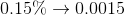### Example Question #9 : How To Find Decimal Equivalent To A Percentage

Find the decimal equivalent to the percentage: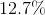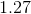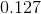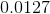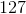Explanation:

In order to find the decimal equivalent of a percentage, the number that makes up the percent has to be divided by 100. However, since it is division by a power of 10, we can accomplish the same thing, by moving the decimal point 2 places to the left, thus making the number smaller. For this problem, that looks like this: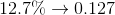### Example Question #10 : How To Find Decimal Equivalent To A Percentage

Find the decimal equivalent of the percentage: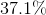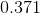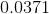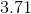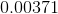Explanation:

In order to find the decimal equivalent of a percentage, the number that makes up the percent has to be divided by 100. However, since it is division by a power of 10, we can accomplish the same thing, by moving the decimal point 2 places to the left, thus making the number smaller. For this problem, that looks like this: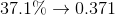← Previous 1

### All SAT Math Resources